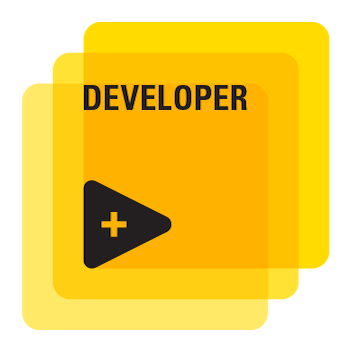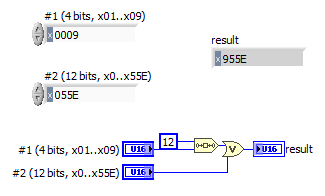# LabVIEW

cancel
Showing results for
Did you mean:

Solved!
Go to solution

In a application I have a requirement to generate a "sum" of two numbers :

Number 1 : 0x01 to 0x09 is the range ( bits 15-12 in final answer )

Number 2 : 0 - 0x55E is the range ( bits 11 - 0 in final answer )

If I have to use 0x01 and 0x0 , I must get an answer of 0x10

If I have to use 0x01 and 0x55E , I must get an answer of 0x155E

If I have to use 0x09 and 0x55E , I must get an answer of 0x955E

Searching for a bit manipulation function in LV ...

Raghunathan
LabVIEW to Automate Hydraulic Test rigs.
Message 1 of 14
(227 Views)

## Re: Adding two Hex values

Does it help for me to emphasize that you are dealing with two high bytes and two low bytes, and data are usually arranged as an array of bytes in memory?

Bill(Mid-Level minion.)
My support system ensures that I don't look totally incompetent.
Proud to say that I've progressed beyond knowing just enough to be dangerous. I now know enough to know that I have no clue about anything at all.
Humble author of the CLAD Nugget.
Message 2 of 14
(204 Views)

## Re: Adding two Hex values

N1×2^12+N2 if N2 is truly 12 bits

You could run N2 through In Range and Coerce to 55E although,  I suspect you meant 7FF?

Ox55E is 0b0101 0101 1110 and that's just a strange limit 7FF would be a max signed I12

What is the data source and LabVIEW datatypes?

For PURE bit manipulations you could also use Split Number and Join Numbers on the math functions pallet.

"Should be" isn't "Is" -Jay
Message 3 of 14
(192 Views)

## Re: Adding two Hex values

@JÞB wrote:

N1×2^12+N2 if N2 is truly 12 bits

You could run N2 through In Range and Coerce to 55E although,  I suspect you meant 7FF?

Ox55E is 0b0101 0101 1110 and that's just a strange limit 7FF would be a max signed I12

What is the data source and LabVIEW datatypes?

For PURE bit manipulations you could also use Split Number and Join Numbers on the math functions pallet.

From the description given, I don't think they want to add anything; it seems they just want the first number to the MSB, while the second number is the LSB, so I think your second solution is the one they want.

Bill(Mid-Level minion.)
My support system ensures that I don't look totally incompetent.
Proud to say that I've progressed beyond knowing just enough to be dangerous. I now know enough to know that I have no clue about anything at all.
Humble author of the CLAD Nugget.
Message 4 of 14
(168 Views)
Solution
Accepted by topic author MogaRaghu

## Re: Adding two Hex values

@MogaRaghu wrote:

In a application I have a requirement to generate a "sum" of two numbers :

Number 1 : 0x01 to 0x09 is the range ( bits 15-12 in final answer )

Number 2 : 0 - 0x55E is the range ( bits 11 - 0 in final answer )

If I have to use 0x01 and 0x0 , I must get an answer of 0x10

If I have to use 0x01 and 0x55E , I must get an answer of 0x155E

If I have to use 0x09 and 0x55E , I must get an answer of 0x955E

When talking about bit manipulations, you need to be very specific defining the problem!

As a first step, let's define the datatype of the inputs. Is #1 U8 or U16? I assume #2 is U16. Is this correct?  Is the result also supposed to be U16? can we assume that none of the unused bits are set?

Let's assume you don't have to "use" any specific input and let's just say these are example input and results.

If you made a typo and the first line: "If I have to use 0x01 and 0x0 , I must get an answer of 0x10" should actually produce an answer of x1000, here's what you can do:(If you need x10 (instead of x1000) as first result, there is no simple solution (because we need to define special handling for some input combos!)! If #1 is U8, you need to convert to U16 first. Let's assume that the inputs are well formed and always in the valid range, else slightly more code is needed to validate the inputs and even possibly generate errors for inputs that are out of range.)LabVIEW Champion.Message 5 of 14
(157 Views)

## Re: Adding two Hex values

@billko wrote:

@JÞB wrote:

N1×2^12+N2 if N2 is truly 12 bits

You could run N2 through In Range and Coerce to 55E although,  I suspect you meant 7FF?

Ox55E is 0b0101 0101 1110 and that's just a strange limit 7FF would be a max signed I12

What is the data source and LabVIEW datatypes?

For PURE bit manipulations you could also use Split Number and Join Numbers on the math functions pallet.

From the description given, I don't think they want to add anything; it seems they just want the first number to the MSB, while the second number is the LSB, so I think your second solution is the one they want.

That's just the way math works

X*Radix^N is the digits of X shifted N places in any representation.

10*10^1=100

19*10^0=19

49*7^-1=7(or 10base7)

*2^12 is a left shift of 12 binary digits OR a shifted value with a value less than 2^N is an add.  Like adding 1500 and 85 gets 1585 in decimal.

So splitting N1 and using its lower nibble as the MSB joined to the 12 bits of N2 is exactly the same as N1*2^12+N2 AND N1<<12 bitwise Or N2 as Altenbach posted.

"Should be" isn't "Is" -Jay
Message 6 of 14
(144 Views)

## Re: Adding two Hex values

That  is the exact function I was searching : Bit Shift.   Thanks Altenbach !!

And thanks to all those who quipped in. When posting a specific part of a problem, it raises lots of basic doubts ...here is the actual requirement : ( Though we have two distinct parts of 4 bits and 12 bits not all are used in the application. Unused places are padded with 0  )Raghunathan
LabVIEW to Automate Hydraulic Test rigs.
Message 7 of 14
(129 Views)

## Re: Adding two Hex values

@JÞB wrote:

@billko wrote:

@JÞB wrote:

N1×2^12+N2 if N2 is truly 12 bits

You could run N2 through In Range and Coerce to 55E although,  I suspect you meant 7FF?

Ox55E is 0b0101 0101 1110 and that's just a strange limit 7FF would be a max signed I12

What is the data source and LabVIEW datatypes?

For PURE bit manipulations you could also use Split Number and Join Numbers on the math functions pallet.

From the description given, I don't think they want to add anything; it seems they just want the first number to the MSB, while the second number is the LSB, so I think your second solution is the one they want.

That's just the way math works

X*Radix^N is the digits of X shifted N places in any representation.

10*10^1=100

19*10^0=19

49*7^-1=7(or 10base7)

*2^12 is a left shift of 12 binary digits OR a shifted value with a value less than 2^N is an add.  Like adding 1500 and 85 gets 1585 in decimal.

So splitting N1 and using its lower nibble as the MSB joined to the 12 bits of N2 is exactly the same as N1*2^12+N2 AND N1<<12 bitwise Or N2 as Altenbach posted.

I never considered that.  Perfect explanation.  THANKS!

Bill(Mid-Level minion.)
My support system ensures that I don't look totally incompetent.
Proud to say that I've progressed beyond knowing just enough to be dangerous. I now know enough to know that I have no clue about anything at all.
Humble author of the CLAD Nugget.
Message 8 of 14
(120 Views)

## Re: Adding two Hex values

@MogaRaghu wrote:

@MogaRaghu wrote:

That  is the exact function I was searching : Bit Shift.   Thanks Altenbach !!

And thanks to all those who quipped in. When posting a specific part of a problem, it raises lots of basic doubts ...here is the actual requirement : ( Though we have two distinct parts of 4 bits and 12 bits not all are used in the application. Unused places are padded with 0  )That  is the exact function I was searching : Bit Shift.   Thanks Altenbach !!

And thanks to all those who quipped in. When posting a specific part of a problem, it raises lots of basic doubts ...here is the actual requirement : ( Though we have two distinct parts of 4 bits and 12 bits not all are used in the application. Unused places are padded with 0  )With the new information you will need additional steps in Altenbachs approach.   N2 should be ANDED with 0xFFF to mask the upper 4 bits then run through In Range and Coerce (0, 1599) and throw a "parameter out of bounds" error if Coerced, Masked N2 is not equal to N2.

Similarly N1 should be tested to be in range (0, 9) and throw an "Invalid Address" error if Coerced? is TRUE.

Then you can avoid sending malformed commands.

"Should be" isn't "Is" -Jay
Message 9 of 14
(111 Views)

## Re: Adding two Hex values

@JÞB wrote:
With the new information you will need additional steps in Altenbachs approach.

Well, my "approach" was also explaining that if the inputs are not "clean", further code is needed and potentially errors generated. (quote from hereLet's assume that the inputs are well formed and always in the valid range, else slightly more code is needed to validate the inputs and even possibly generate errors for inputs that are out of range.")

For completeness, here's the forward and reverse operation (Still assuming that the original inputs are clean!).@JÞB wrote:
N2 should be ANDED with 0xFFF to mask the upper 4 bits then run through In Range and Coerce (0, 1599) to test for a "parameter out of bounds" error if Coerced? is TRUE.

That seems redundant and dangerous. If we do the "in range" test, the masking is obviously not needed. In fact the ANDing first would mask certain out of range values for #2 and would let errors slip through undetected.

Testing for input errors would make the code significantly less efficient. 😄LabVIEW Champion.Message 10 of 14
(103 Views)